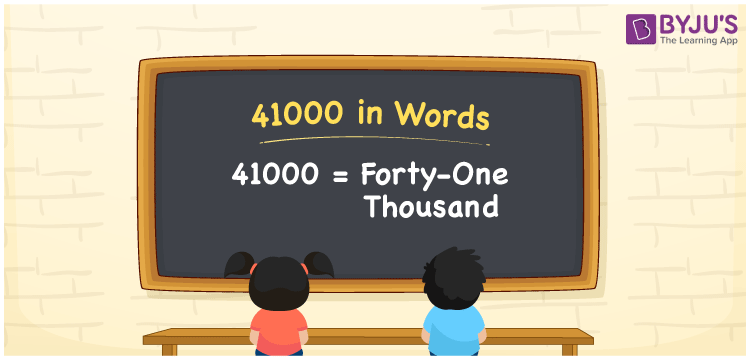# 41000 in Words

We can express 41000 in words as Forty-one thousand. Suppose your monthly salary is Rs. 41000, and this can be conveyed as “My monthly salary is Forty-one thousand rupees”. We can use the place value chart to express the number 41000 in words. Let’s learn how to write the cardinal number 41000 in words.

 41000 in words Forty-one thousand Forty-one thousand in Numbers 41000

## 41000 in English Words

Using the English alphabet, we generally write numbers in words. Thus, we can read 41000 in English as “Forty-one thousand”.## How to Write 41000 in Words?

As 41000 contains five digits, we should make a place chart with 5 columns as shown below.

 Ten thousands Thousands Hundreds Tens Ones 4 1 0 0 0

This can be written as:

4 × Ten thousand + 1 × Thousand + 0 × Hundred + 0 × Ten + 0 × One

= 4 × 10000 + 1 × 1000 + 0 × 100 + 0 × 10 + 0 × 1

= 40000 + 1000

= 41000

= Forty-one thousand

Therefore, 41000 in words is written as Forty-one thousand.

In the above place value chart, ones = 0, tens = 0, hundreds = 0, thousands = 1, ten thousands = 4. Thus, and by combining the numbers at thousands and ten thousand places, we get Forty-one thousand since 4 × 10 = 40, i.e. forty and 40 + 1 = 41, i.e. forty-one.

41000 is a natural number that precedes 41001 and succeeds 40999.

41000 in words – Forty-one thousand

Is 41000 an odd number? – No

Is 41000 an even number? – Yes

Is 41000 a perfect square number? – No

Is 41000 a perfect cube number? – No

Is 41000 a prime number? – No

Is 41000 a composite number? – Yes

## Frequently Asked Questions on 41000 in Words

Q1

### How do you write 41000 in words?

41000 can be written in English words as Forty-one thousand.
Q2

### How do you write 41000 in words on a cheque?

On a cheque, we generally write 41000 in words as Forty-one thousand rupees only.
Q3

### Write 41000 in expanded form.

The expanded form of the number 41000, i.e. Forty-one thousand is: 41000 = 40000 + 1000 Or 41000 = 4 × 10000 + 1 × 1000 + 0 × 100 + 0 × 10 + 0 × 1